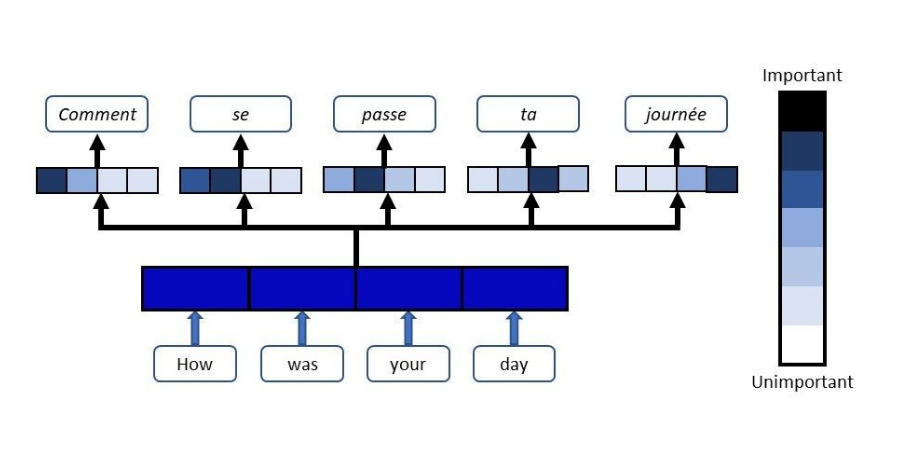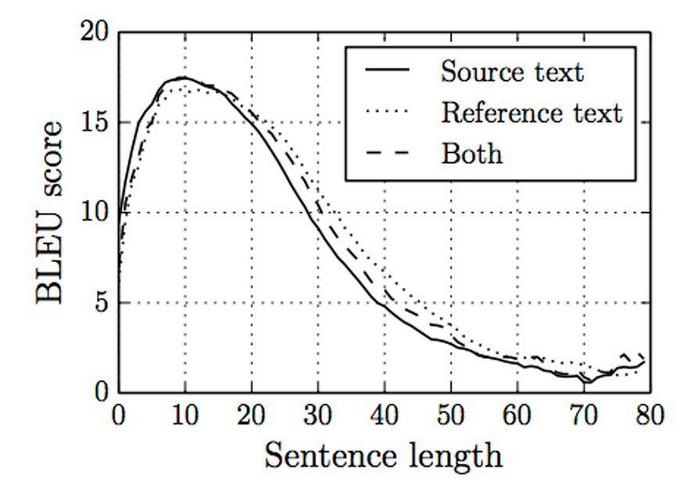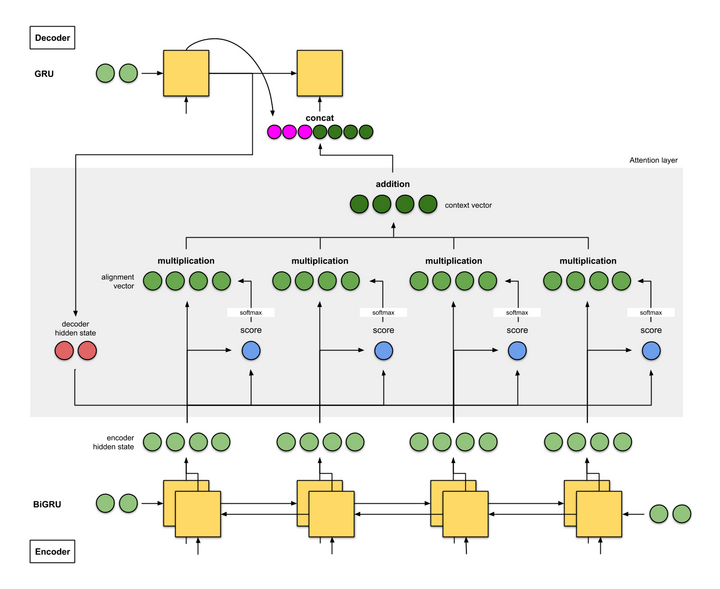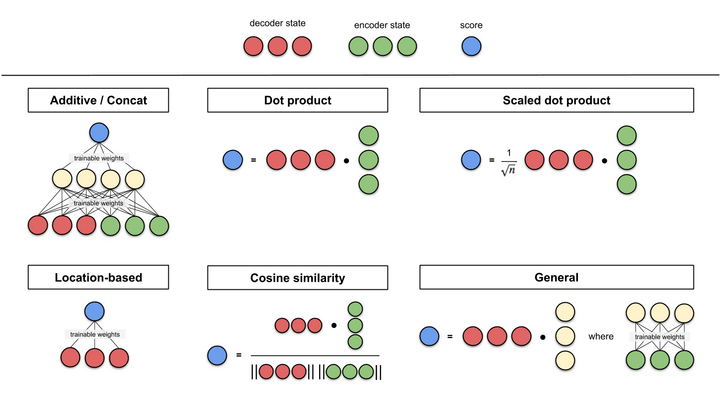# Attention MechanismS.No Lesson Title
1
2
3
##### Intuition behind Attention Mechanism
3.1 Flow of Data in Attention Mechanism
3.2 Implementation of Attention Layers
4

### Introduction

The idea of Attention Mechanism has changed the entire landscape of Natural Language Processing. It led to the development of transformers and many similar architectures/models which have left behind LSTM/GRU's in their performance. Attention mechanisms were already used in the field of visual imaging in the 1990s. The first paper to implement this idea for Natural Language Processing was "Neural Machine Translation by Jointly Learning to Align and Translate" by Dzmitry Bahdanau. This paper was the base of many other studies related to using Attention Mechanisms for NLP tasks.

The next big breakthrough came when Google's machine translation team used self-attention to learn text representation in their paper "Attention is All You Need". This paper didn't use attention with RNN but they introduced a whole new architecture called transformer based on parallel learning compared to the idea of sequential learning in RNN's. Transformers have revolutionized the field of Natural Language Processing and the idea of powering them is of Self- Attention. In this article, we'll mainly focus on basic Attention Mechanisms and try to understand how they have led to new architectures in the field of Natural Language Processing.

### Need for Attention Mechanism

To understand the idea behind the attention mechanism we'll take the example of an encoder-decoder model. The basic idea behind encoder-decoder is that encoder takes an input sequence and represents/encodes it in the form of a vector. This vector called the context vector is given to the decoder, which then generates another targeted sequence in decoded form. One example of usage of encoder-decoder is language translation where one language is encoded by an encoder and then decoded by the decoder to the target language.

Now the problem arises when the input sequence is very long. In that case, the single vector from the encoder doesn't provide enough information for the decoder to construct the target sentence. Have a look at the following figure which shows how the performance of the sequential encoder-decoder without attention mechanism keeps decreasing with an increase in sentence length.BLEU (Bilingual Evaluation Understudy) is a score used to compare the translation of a text to one or more reference candidates. From the above figure, we can see that encoder-decoders without attention work well for shorter sentences but their memorization power goes down when sentence length increases. The attention mechanism gives more contextual information to the decoder. It informs the decoder how much "attention" it should give to each input word at every decoding step.

### Intuition behind Attention Mechanism

The simplest way to think about the core idea behind the attention mechanism is that every time the decoder predicts an output word, it only looks at a part of the input where the most important information is present instead of looking at the entire sequence in compressed form.

#### Flow of Data in Attention Mechanism

Attention acts as an interface connecting the encoder and the decoder providing the decoder with information from every hidden state of the encoder. This helps the decoder to learn the association between different parts of the sentence thus helping it to cope with long input sentences. Have a look at the following model architecture having bi-directional RNN/LSTM/GRU with an attention layer.In the figure above the attention given to words is based on the window size (T) of four words. The input sequence is fed into the encoder whose hidden states are exposed to the decoder via the attention layer. Since we have a bi-directional layer the forward and backward hidden states are concatenated. These states are then passed on to the attention layer and weighted to give a context vector. Attention weights or scores are calculated by aligning the decoder's last hidden state with the encoder's hidden states.

In short, the decoder's current hidden state is a function of it's previous hidden state, previous output word, and the context vector. Attention to the decoder is passed via the context vector which depends on the alignment of decoder and encoder hidden states. Now let's see what alignment means and how the flow of values takes place. Following is a figure which shows the different alignment scoring functions to calculate the score.The alignment score is used to quantify how well the output at position i is aligned to the input at position j. You can see that the context vector that is passed on to the decoder is a weighted sum of 4 different encoders hidden stated. The weights (or alignment score) used here comes from the alignment method used. We can create a standard method to calculate the alignment energy e, alignment weight α, context vector c given the alignment model a:

eij = a(si-1,hj)

Αij = exp exp (eij) Σ T k=1 exp exp(eik)

ci = Σ T i=1 Αijhj

The decoder's hidden state depends on its previous hidden state , the previous predicted word, and the current context vector. At each time step, the context vector is adjusted using the alignment model and attention. Thus at each decoder time step, a selective set of inputs are attended to by the decoder. Now we'll have a look at a step-by-step approach using all the above equations.

Let's take an example where a model reads the English(input language) sentence from start till end, after which it starts translating to Spanish (the output language). While translating each English word, it makes use of the keywords it has understood with the help of an attention mechanism.

Attention places different focus on different words by assigning each word with a score. Then, using the softmax scores, we aggregate the encoder hidden states using a weighted sum of the encoder hidden states to get the context vector.

#### Implementation of Attention Layers

The implementations of an attention layer can be broken down into 4 steps.

Step 0: Prepare hidden states

First, prepare all the available encoder hidden states (green) and the first decoder hidden state (red). In our example, we have 4 encoder hidden states and the current decoder hidden state. (Note: the last consolidated encoder hidden state is fed as input to the first time step of the decoder. The output of this first-time step of the decoder is called the first decoder hidden state.)

Step 1: Obtain a score for every encoder hidden state

A score (scalar) is obtained by a score function (also known as the alignment score function or alignment model). In this example, the score function is a dot product between the decoder and encoder hidden states.

Step 2: Run all the scores through a Softmax layer

We put the scores to a softmax layer so that the softmax scores (scalar) add up to 1. These softmax scores represent the attention distribution.

Step 3: Multiply each encoder hidden state by its softmax score

By multiplying each encoder's hidden state with its softmax score (scalar), we obtain the alignment vector or the annotation vector. This is exactly the mechanism where alignment takes place.

Step 4: Sum the alignment vectors

The alignment vectors are summed up to produce the context vector. A context vector is an aggregated information of the alignment vectors from the previous step.

Step 5: Feed the context vector into the decoder

This is the simplest approach when it comes to building an attention mechanism for Natural Language Processing Models. All the other advanced methods are derived out from this by tweaking the idea of how attention is calculated.

### Conclusion

In this article, we have covered how attention mechanisms came into the picture and how the first set of models looked like. There are many other advanced models which have led to improvement in the basic idea of attention we have discussed. You can always read about them and you can see how they build upon the idea of attention we have discussed in this article. One good place to start is the paper "Attention is All You Need" which gave birth to transformers. Happy Learning!